# Series-Parallel DC Circuits

## DC Electric Circuits

• #### Question 1

 Don’t just sit there! Build something!!

Learning to mathematically analyze circuits requires much study and practice. Typically, students practice by working through lots of sample problems and checking their answers against those provided by the textbook or the instructor. While this is good, there is a much better way.

You will learn much more by actually building and analyzing real circuits, letting your test equipment provide the “answers” instead of a book or another person. For successful circuit-building exercises, follow these steps:

1. Carefully measure and record all component values prior to circuit construction.
2. Draw the schematic diagram for the circuit to be analyzed.
3. Carefully build this circuit on a breadboard or other convenient medium.
4. Check the accuracy of the circuit’s construction, following each wire to each connection point, and verifying these elements one-by-one on the diagram.
5. Mathematically analyze the circuit, solving for all values of voltage, current, etc.
6. Carefully measure those quantities, to verify the accuracy of your analysis.
7. If there are any substantial errors (greater than a few percent), carefully check your circuit’s construction against the diagram, then carefully re-calculate the values and re-measure.

Avoid very high and very low resistor values, to avoid measurement errors caused by meter “loading”. I recommend resistors between 1 kΩ and 100 kΩ, unless, of course, the purpose of the circuit is to illustrate the effects of meter loading!

One way you can save time and reduce the possibility of error is to begin with a very simple circuit and incrementally add components to increase its complexity after each analysis, rather than building a whole new circuit for each practice problem. Another time-saving technique is to re-use the same components in a variety of different circuit configurations. This way, you won’t have to measure any component’s value more than once.

• #### Question 2

In a series circuit, certain general rules may be stated with regard to quantities of voltage, current, resistance, and power. Express these rules, using your own words:

“In a series circuit, voltage . . .”

“In a series circuit, current . . .”

“In a series circuit, resistance . . .”

“In a series circuit, power . . .”

For each of these rules, explain why it is true.

• #### Question 3

From observation of this circuit (with components attached to a “terminal strip”), draw an appropriate schematic diagram: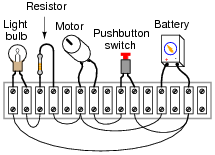• #### Question 4

In a parallel circuit, certain general rules may be stated with regard to quantities of voltage, current, resistance, and power. Express these rules, using your own words:

Ïn a parallel circuit, voltage . . .”

Ïn a parallel circuit, current . . .”

Ïn a parallel circuit, resistance . . .”

Ïn a parallel circuit, power . . .”

For each of these rules, explain why it is true.

• #### Question 5

Calculate the resistance between points A and B (RAB) for the following resistor networks:• #### Question 6

Complete the table of values for this circuit:• #### Question 7

Complete the table of values for this circuit:• #### Question 8

Complete the table of values for this circuit:• #### Question 9

Complete the table of values for this circuit:• #### Question 10

Identify which of these components are connected directly in series with each other, and which are connected directly in parallel with each other:Assume that the open wire ends are connection points to a power source.

• #### Question 11

Identify which of these components are connected directly in series with each other, and which are connected directly in parallel with each other:Assume that the open wire ends are connection points to a power source. In circuits where ground symbols appear, consider ground as the other side of the power source.

• #### Question 12

Identify which of these components are connected directly in series with each other, and which are connected directly in parallel with each other: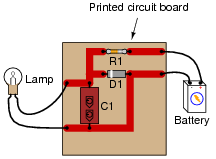• #### Question 13

Rank these three light bulb assemblies according to their total electrical resistance (in order of least to greatest), assuming that each of the bulbs is the same type and rating:Explain how you determined the relative resistances of these light bulb networks.

• #### Question 14

Which components are guaranteed to share the exact same voltage by virtue of their connections with each other? Which components are guaranteed to share the exact same current by virtue of their connections with each other?• #### Question 15

Which components in this partial automobile schematic diagram are guaranteed to share the exact same voltage by virtue of their connections with each other? Which components are guaranteed to share the exact same current by virtue of their connections with each other?• #### Question 16

In this series-parallel circuit, resistors R1 and R2 are in series with each other, but resistor R3 is neither in series nor in parallel with either R1 or R2:Normally, the first step in mathematically analyzing a circuit such as this is to determine the total circuit resistance. In other words, we need to calculate how much resistance the voltage source “sees” in the network formed by R1, R2, and R3. If the circuit were a simple series configuration, our task would be easy:Likewise, if the circuit were a simple parallel configuration, we would have no difficulty at all calculating total resistance:Due to the fact that our given circuit is neither purely series nor purely parallel, though, calculation of total resistance is not a simple one-step operation. However, there is a way we could simplify the circuit to something that is either simple series or simple parallel. Describe how that might be done, and demonstrate using numerical values for resistors R1, R2, and R3.

• #### Question 17

Rank these five light bulb assemblies according to their total electrical resistance (in order of least to greatest), assuming that each of the bulbs is the same type and rating:Explain how you determined the relative resistances of these light bulb networks.

• #### Question 18

Determine the amount of electrical resistance indicated by an ohmmeter connected between the following points in this circuit: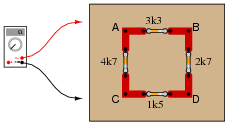• Between points A and B =
• Between points A and C =
• Between points C and D =
• Between points D and B =
• Between points B and C =

Explain whether or not it makes any sense to speak of a “total” resistance for this network.

• #### Question 19

Calculate the amount of voltage dropped across resistor R2:Also, note the direction of current through it and the polarity of the voltage drop across it.

• #### Question 20

Antique American automobiles often used 6 volt electrical systems instead of the 12 volt components found in more modern cars and trucks. People who restore these old vehicles may have difficulty finding old 6-volt generators and batteries to replace the defective, original units. An easy solution is to update the vehicle’s generator and battery with modern (12 volt) components, but then another problem arises.

A 12 volt generator and 12 volt battery will overpower the old 6 volt headlights, brake lights, and other electrical “loads” in the vehicle. A solution used by antique automobile restorers is to connect resistors between the 12-volt generator system and the 6-volt loads, like this:Explain why this solution works, and also discuss some of the disadvantages of using resistors to adapt the new (12 volt) to the old (6 volt) components.

• #### Question 21

Draw a schematic diagram of this “breadboarded” circuit: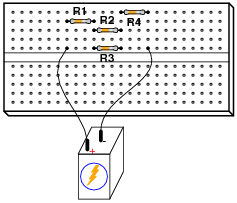• #### Question 22

Think of a way to re-wire the electrical system of this old automobile (with 6-volt light bulbs) so as to not require resistors between the loads and the generator/battery portion of the circuit (operating at 12 volts each).• #### Question 23

Calculate the voltage drops VAB, VBC, and VCD in the following circuit: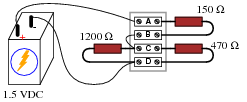• #### Question 24

Calculate the voltage magnitude and polarity between points A and D in this circuit, assuming a power supply output voltage of 10.5 volts: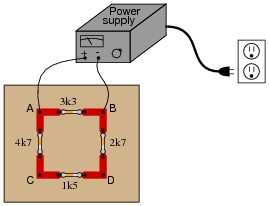Also, calculate the total current output by the power supply as it energizes this resistor network.

• #### Question 25

Calculate the power supply’s output (total) current: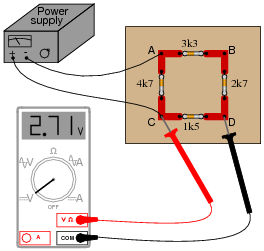• #### Question 26

Complete the table of values for this circuit:• #### Question 27

Complete the table of values for this circuit:• #### Question 28

Complete the table of values for this circuit: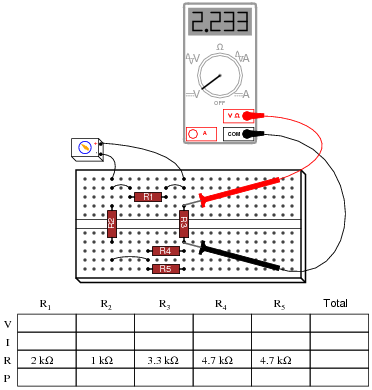• #### Question 29

What would happen to the voltage drops across each resistor in this circuit if resistor R1 were to fail open?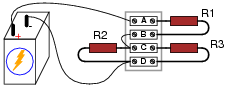• #### Question 30

What would happen to the voltage drops across each resistor in this circuit if either resistor R2 or R3 were to fail open?• #### Question 31

What will happen to each resistor’s voltage and current in this circuit if resistor R1 fails open? Provide individual answers for each resistor, please.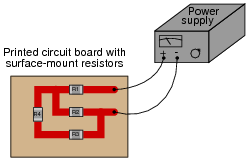• #### Question 32

What will happen to each resistor’s voltage and current in this circuit if resistor R2 fails shorted? Provide individual answers for each resistor, please.• #### Question 33

What will happen to each resistor’s voltage in this circuit if resistor R4 fails shorted? Provide individual answers for each resistor, please.Also, comment on the practical likelihood of a resistor failing shorted, as opposed to failing open.

• #### Question 34

A student built this resistor circuit on a solderless breadboard, but made a mistake positioning resistor R3. It should be located one hole to the left instead of where it is right now: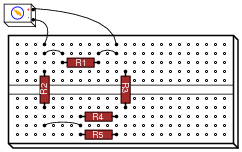Determine what the voltage drop will be across each resistor, in this faulty configuration, assuming that the battery outputs 9 volts.

• R1 = 2 k Ω VR1 =
• R2 = 1 k Ω VR2 =
• R3 = 3.3 k Ω VR3 =
• R4 = 4.7 k Ω VR4 =
• R5 = 4.7 k Ω VR5 =
• #### Question 35

Suppose you were designing a circuit that required two LEDs for “power on” indication. The power supply voltage is 15 volts, and each LED is rated at 1.6 volts and 20 mA. Calculate the dropping resistor sizes and power ratings:After doing this, a co-worker looks at your circuit and suggests a modification. Why not use a single dropping resistor for both LEDs, economizing the number of components necessary?Re-calculate the dropping resistor ratings (resistance and power) for the new design.

• #### Question 36

Calculate all voltages and currents in this circuit: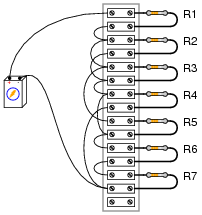The battery voltage is 15 volts, and the resistor values are as follows:

R1 = 1 kΩ
R2 = 3.3 kΩ
R3 = 4.7 kΩ
R4 = 2.5 kΩ
R5 = 10 kΩ
R6 = 1.5 kΩ
R7 = 500 Ω
• #### Question 37

Examine these two variable-resistance (rheostat) networks, each one with a large-range potentiometer and a small-range potentiometer:For each network, determine which pot is the coarse adjustment and which pot is the fine adjustment for total resistance.

• #### Question 38

Identify which of these components are connected directly in series with each other, and which are connected directly in parallel with each other: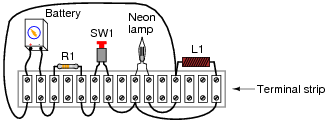• #### Question 39

Complete the table of values for this circuit:• #### Question 40

Complete the table of values for this circuit:• #### Question 41

Determine which light bulb(s) will glow brightly, and which light bulb(s) will glow dimly (assuming all light bulbs are identical).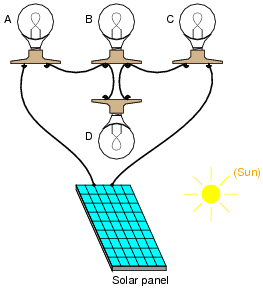### Related Content

• Share

Published under the terms and conditions of the Creative Commons Attribution License•Alana Ben May 19, 2020

How did you guys find the answer for Q5, figure 6

Like.
•The Mullet May 26, 2020
I don't know if there is an easier way, but I was able to calculate the resistance using the delta-y conversion for a bridge resistor circuit. https://www.allaboutcircuits.com/textbook/direct-current/chpt-10/delta-y-and-y-conversions/
Like.
•RK37 May 28, 2020
The 330 Ω and 470 Ω resistors are in parallel; they don't look like they're in parallel, but note that their terminals are connected to the same two nodes. REQ for those two resistors (call it REQ1) is ~193.9 Ω. If you redraw the circuit with REQ1, there are two (parallel) current paths between node A and node B: one consisting of the 220 Ω resistor and REQ1 in series, and the other consisting of the 100 Ω resistor. REQ2 = REQ1 + 220 Ω = 413.9 Ω, and then RAB = 100 Ω || REQ2 = 80.54 Ω.
Like.
• K
kubajs June 04, 2020
Do you guys know how to calculate the resistance in case we add another resistor between the B point and 470 ohm resistor? Something like this: http://tinyurl.com/y8n3kdfe I cannot figure out the solution.
Like.
• K
kubajs June 05, 2020
Looks like Y-Δ_transform might be solution for this. Haven't studied it yet so I need to take a look :).
Like.
•RK37 June 09, 2020
You're correct, the delta/wye transform is the preferred approach for calculating an equivalent resistance in the circuit configuration that you're describing. You can read about it here: https://www.allaboutcircuits.com/technical-articles/delta-wye-transforms-behind-the-scenes/
Like.